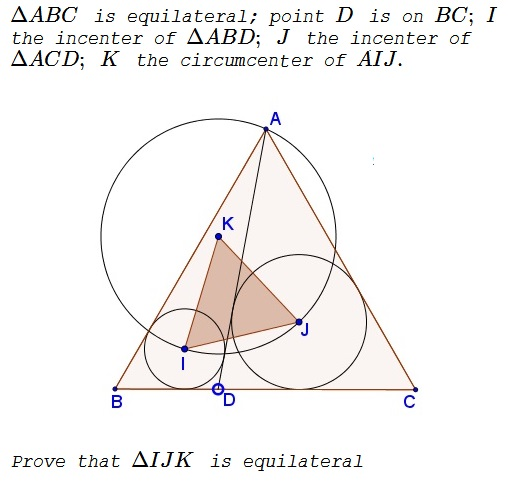# Van Khea's Quickie

### Problem### Solution

$AI\,$ is the bisector of $\angle BAD,\,$ $AJ\,$ is the bisector of $\angle CAD.\,$ Since $\angle BAC=60^{\circ},\,$ $\angle IAJ=30^{\circ}.\,$But $\angle IAJ\,$ is inscribed into circle $(K),\,$ implying that it is subtended by the arc $\overset{\frown}{AJ}=60^{\circ}.\,$ The corresponding central $\angle IKJ=60^{\circ},\,$ and, since $\Delta IKJ\,$ is isosceles $(IK=JK),\,$ it is also equilateral.

### Acknowledgment

The above problem was posted by Van Khea (Cambodia) at the Olimpiada pe Şcoală (The School Yard Olympiad) and commented with the above solution by Marian Dincă (Romania).

• Angle Trisectors on Circumcircle
• Equilateral Triangles On Sides of a Parallelogram
• Pompeiu's Theorem
• Pairs of Areas in Equilateral Triangle
• The Eutrigon Theorem
• Equilateral Triangle in Equilateral Triangle
• Seven Problems in Equilateral Triangle
• Spiral Similarity Leads to Equilateral Triangle
• Parallelogram and Four Equilateral Triangles
• A Pedal Property in Equilateral Triangle
• Miguel Ochoa's van Schooten Like Theorem
• Two Conditions for a Triangle to Be Equilateral
• Incircle in Equilateral Triangle
• When Is Triangle Equilateral: Marian Dinca's Criterion
• Barycenter of Cevian Triangle
• Excircle in Equilateral Triangle
• Converse Construction in Pompeiu's Theorem
• Wonderful Trigonometry In Equilateral Triangle
• 60o Angle And Importance of Being The Other End of a Diameter
• One More Property of Equilateral Triangles
• Equilateral Triangle from Three Centroids
•